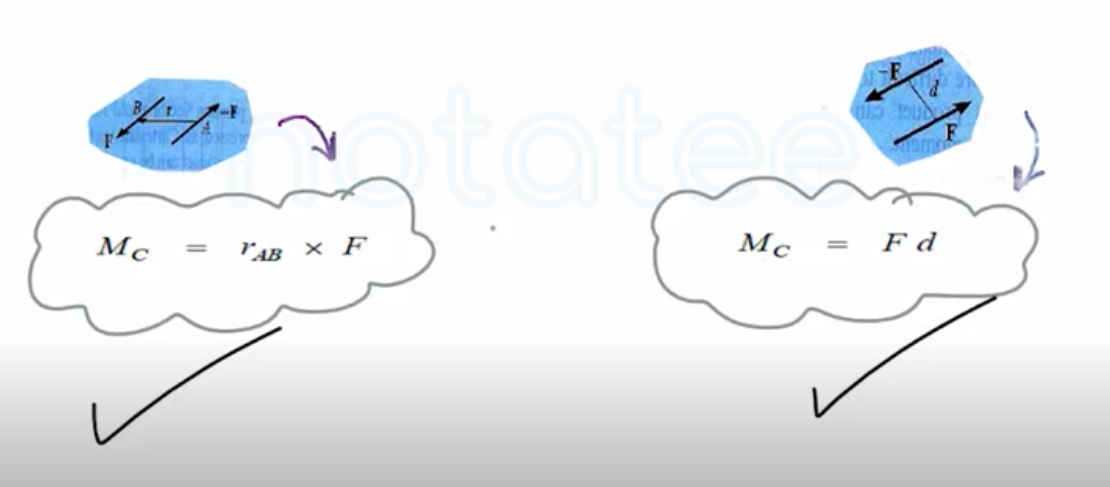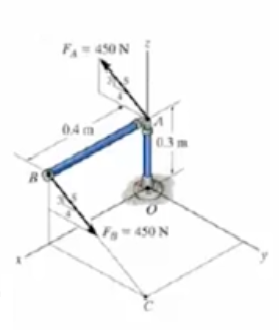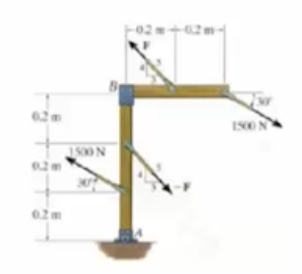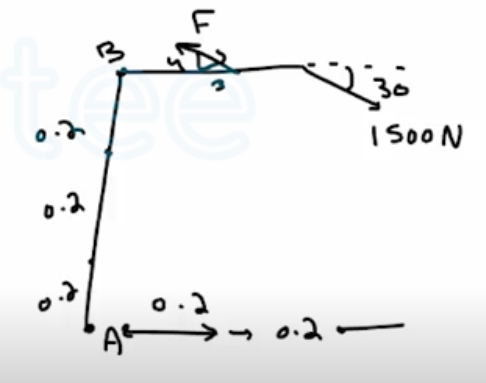Need Help?

Subscribe to Statics

###### \${selected_topic_name}
• Notes

Couple MomentDetermine the couple moment acting on the pipe assembly and express the result as a Cartesian vector.$F_{A}=[0i-450(\frac{4}{5})j=450(\frac{3}{5})k]N$

$=(0 i-360\hat j+270 k ) N$

$M_{c}=\vec{r}_{B A} \times \overrightarrow F_{A}$

$=\left|\begin{array}{ccc}{i} & {j} & {k} \\ {-0.4} & {0} & {0} \\ {0-360} & {+270}\end{array}\right|= (o \hat i+108 \hat j+144 k) N m$

Determine the required magnitude of force  $\mathbf{F}$  if the  resultant couple moment on the frame is  $200 \mathrm{N} \cdot \mathrm{m},$ clockwise.$\sum M_{1500}=(1500 \cos 30)(0.4)$

$+(1500 \sin 30)(0.4)$

$=820 N \cdot m$

$M_{F}=F\left(\frac{3}{5}\right)(0.2)+F\left(\frac{4}{5}\right)(0.2).$

$=0.28 \mathrm{F}$

$M_{1500}-M_{F}=200\longrightarrow 820-0.28F=200$

$∴ F=2214 N=2.21 \mathrm{kN}$